Next: 13.1 Regular Polyhedra
Up: Part II: Three-Dimensional Geometry
Previous: 12.3 Lines

# 13 Polyhedra

For any polyhedron topologically equivalent to a sphere---in particular, for any convex polyhedron---the Euler formula holds: v-e+f=2, where v is the number of vertices, e is the number of edges, and f is the number of faces.

Many common polyhedra are particular cases of cylinders (Section 13.2) or cones (Section 13.3). A cylinder with a polygonal base (directrix) is called a prism. A cone with a polygonal base is called a pyramid. A frustum of a cone with a polygonal base is called a trucated pyramid. Formulas (13.2.1) , (13.3.1) and (13.3.2) give the volume of a general prism, pyramid, and trucated pyramid.

A prism whose base is a parallelogram is a parallelepiped. The volume of a parallelepiped with one vertex at the origin and adjacent vertices at (x,y,z), (x,y,z) and (x,y,z) is given byThe rectangular parallelepiped is a particular case: all its faces are rectangles. If the side lengths are a, b, c, the volume is abc, the total area is 2( ab+ac+bc), and each diagonal has length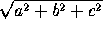. When a=b=c we get the cube: see Section 13.1.

A pyramid whose base is a triangle is a tetrahedron. The volume of a tetrahedon with one vertex at the origin and the other vertices at (x,y,z), (x,y,z) and (x,y,z) is given by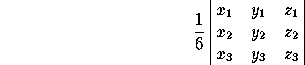In a tetrahedron with vertices P, P, P, P, let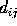be the distance (edge length) from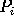to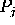. Form the determinantsThen the volume of the tetrahedron is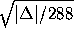, and the radius of the circumscribed sphere is.

Next: 13.1 Regular Polyhedra
Up: Part II: Three-Dimensional Geometry
Previous: 12.3 LinesThe Geometry Center Home Page

Silvio Levy
Wed Oct 4 16:41:25 PDT 1995

This document is excerpted from the 30th Edition of the CRC Standard Mathematical Tables and Formulas (CRC Press). Unauthorized duplication is forbidden.